• 5-DOF机械臂课程设计（二）之视觉引导
千次阅读
2022-02-07 10:03:16

## 相机内参标定

将世界坐标系固定在棋盘上，则棋盘格上的任一点的物理坐标W=0，标定板上的每一个格子大小固定，可以得到每个角点在世界坐标系下的物理坐标 (U, V, W = 0) 。通过这些坐标利用最小二乘法计算出内参矩阵和外参矩阵的积，再依次计算出内参矩阵和外参矩阵。

已知矩阵 H = A ( R 1 R 2 T ) H=A\left(\begin{array}{lll}R 1 & R 2 & T\end{array}\right) , 利用 R 1 , R 2 R 1, R 2 的单位正交关系, 可得
R 1 T R 2 = 0 R 1 T R 1 = R 2 T R 2 = 1 \begin{gathered} R_{1}^{T} R_{2}=0 \\ R_{1}^{T} R_{1}=R_{2}^{T} R_{2}=1 \end{gathered}
可得
H 1 T A − T A − 1 H 2 = 0 H 1 T A − T A − 1 H 1 = H 2 T A − T A − 1 H 2 = 1 \begin{gathered} H 1^{T} A^{-T} A^{-1} H 2=0 \\ H 1^{T} A^{-T} A^{-1} H 1=H 2^{T} A^{-T} A^{-1} H 2=1 \end{gathered}
用矩阵 A A 表示矩阵 B B , 可得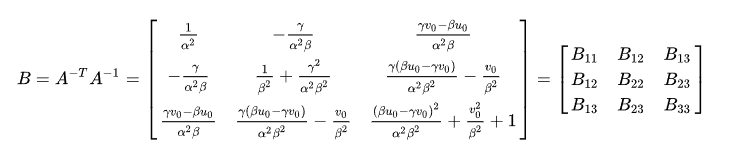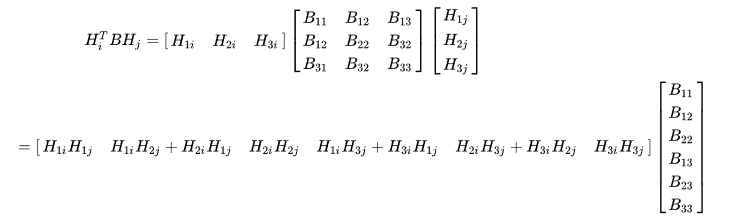​ 只要求解出向量 b ，即可得到矩阵 B 。每张标定板图片可以提供一个vb=0 的约束关系，该约束关系含有两个约束方程。但是，向量 b 有6个未知元素。因此，单张图片提供的两个约束方程是不足以解出来向量 b。因此，我们只要取3张标定板照片，得到3个b的约束关系，即6个方程，即可求解向量 b。当标定板图片的个数大于3时（事实上一般需要15到20张标定板图片），可采用最小二乘拟合最佳的向量 b ，并得到矩阵B。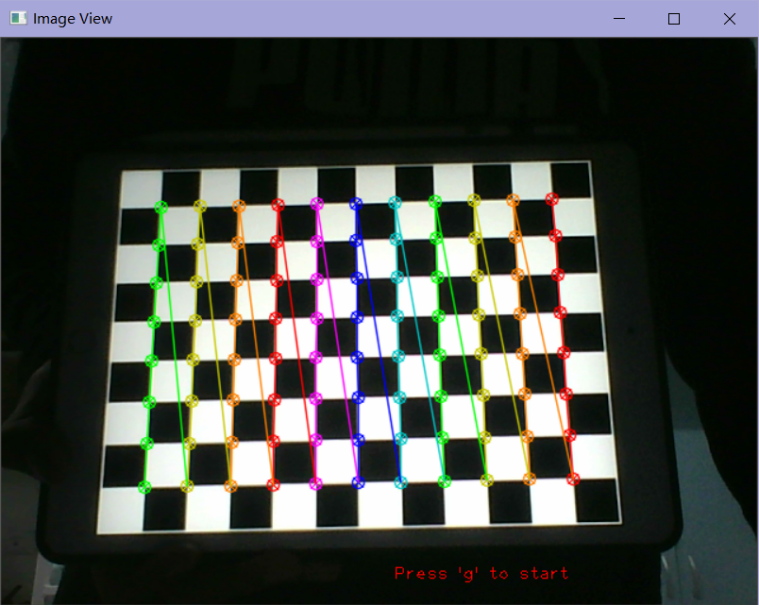## 手眼标定

机器人基座与目标之间位置相对固定的关系建立以下等式:
e n d r 1 T ⋅ r 1 c a m 1 T ⋅ c a m 1 o b j T = e n d r 2 T ⋅ r 2 c a m 2 T ⋅ c a m 2 o b j T { }_{e n d}^{r 1} T \cdot{ }_{r 1}^{c a m 1} T \cdot{ }_{c a m 1}^{o b j} T={ }_{e n d}^{r 2} T \cdot{r 2}^{c a m 2} T \cdot{c a m 2}^{o b j} T
对上式进行变换:
e n d r 2 T − 1 ⋅ e n d r 1 T ⋅ r 1 c a m 1 T = r 2 c a m 2 T ⋅ c a m 2 o b j T ⋅ ⋅ c a m 1 o b j T { }_{e n d}^{r 2} T^{-1} \cdot{ }_{e n d}^{r 1} T \cdot{ }_{r 1}^{c a m 1} T={ }_{r 2}^{c a m 2} T \cdot{ }_{c a m 2}^{o b j} T \cdot{\cdot c a m 1}^{o b j} T
由于相机与末端之间的坐标关系不变, 可令
r 1 c a m 1 T = r 2 c a m 2 T = X { }_{r 1}^{c a m 1} T={ }_{r 2}^{c a m 2} T=X
上述式子可写成:
( r 2 e n d T − 1 ⋅ e n d r 1 T ) ⋅ X = X ⋅ ( o b j c a m 2 T ⋅ c a m 1 o b j T ) 2 \left(\begin{array}{l} r 2 \\ e n d \end{array} T^{-1} \cdot_{e n d}^{r 1} T\right) \cdot X=X \cdot\left(\underset{c a m 2}{o b j} T \cdot{c a m 1}^{o b j} T\right)_{2}^{}
​ 使用OpenCV中的calibrateHandEye函数来实现手眼标定过程，只需要用机械臂获取不同关节角度下识别物体的位姿，得到六组工具坐标系相对于固定坐标系的笛卡尔坐标和对应的六组物体相对于相机的笛卡尔坐标作为输入参数文件，编写程序读取坐标组进行处理转化成旋转矩阵和平移向量，传入calibrateHandEye函数中得到手眼标定矩阵。

## 视觉引导

​ 由于方块具有较为鲜明的颜色特征，所以基于颜色空间进行分割最容易提取出其特征并进行识别提取。但是颜色特征在不同光照强度下，像素值会发生一定波动，所以如下图所示，使用Matlab分析对物块在不同光照强大下进行颜色空间特征分析。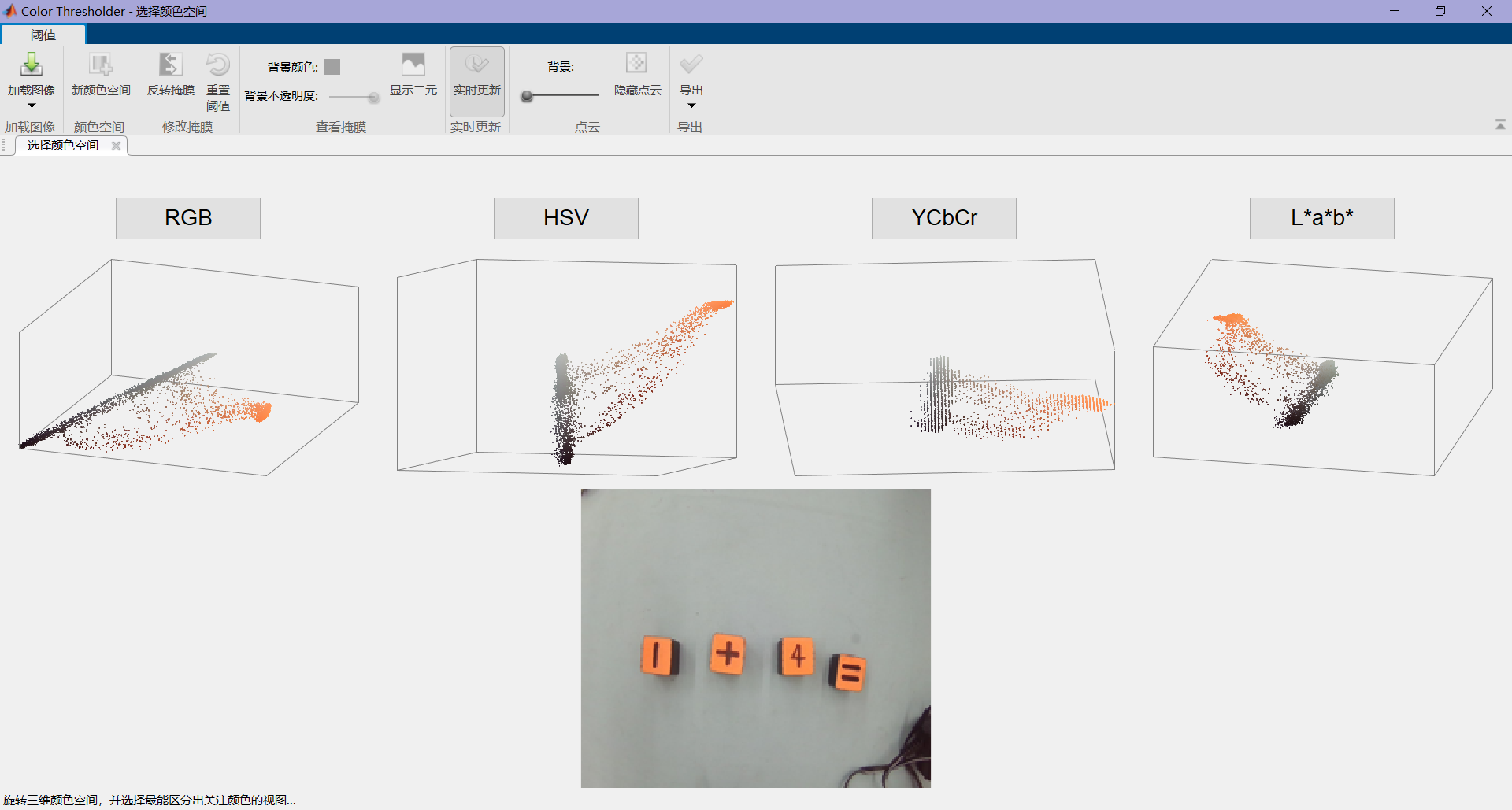​ 使用Matlab的Color Thresholder模块可以很方便的对图像进行不同颜色空间上的特征分析，如下图，可以看到图像的像素值在不同色彩空间下空间特征分布。观察空间特征分布，选取易于进行空间平面分割的色彩空间，这里选择RGB色彩空间进行分割，如下图。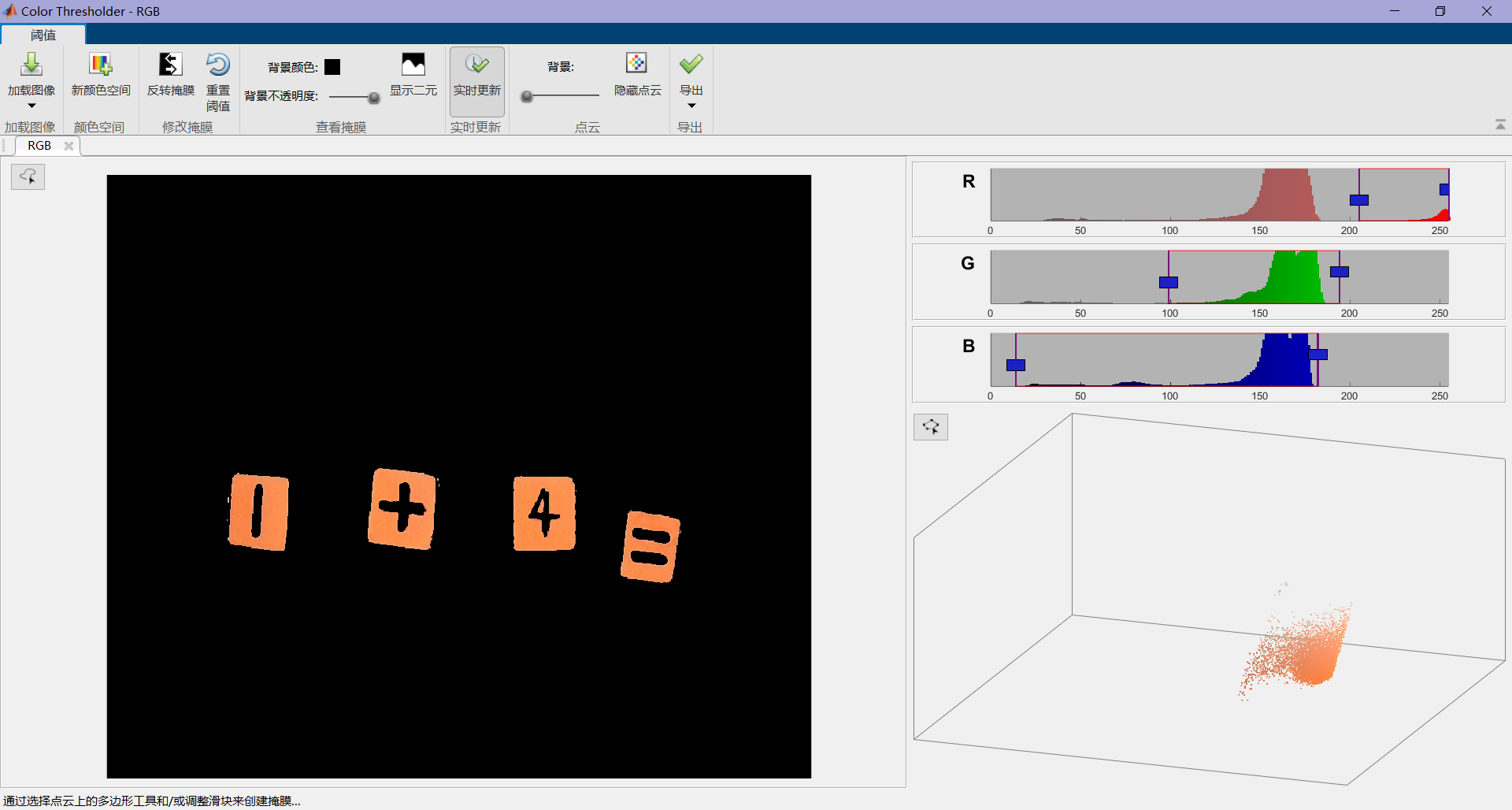​ 通过调整滑块进行色彩空间分割，直到将感兴趣色彩完全从图像中提取出来后，创建掩膜并导出函数，即可得到色彩空间上分割范围。对不同光照强度下相机捕获到的物块图像进行上述分析，取色彩空间范围的并集，即可满足在不同环境下对物块进行识别。

​ 对提取出的掩膜进行图像二值化处理后，提取轮廓并对轮廓进行特征筛选即可获得物块的最小旋转外接矩形，实现对物块的识别。

​ 基于上述结果, 当物块进入到机械臂上相机的视场时, 我们可以计算得到物体相 对于相机的位姿 c a m o b j T { }_{c a m}^{o b j} T 和相机坐标系相对于工具坐标系的变换矩阵 gripper  T { }_{\text {gripper }} T , 已知机械 臂上各关节的角度, 可以通过正运动学得到工具坐标系相对于固定坐标系的变换矩阵 gripper T T , 则有 base
ba  o b j P  base  = gripper ⁡  base  T ⋅ gripper  cam  T ⋅ cam  o b j P \underset{\text { base }}{\stackrel{o b j}{\text { ba }} P}=\underset{\text { base }}{\operatorname{gripper}} T \cdot{ }_{\text {gripper }}^{\text {cam }} T \cdot{ }_{\text {cam }}^{o b j} P
根据机械臂的结构和尺寸, 选择适当的夹取位姿, 得到夹取时末端的 RPY 角, 结 合 base  o b j P { }_{\text {base }}^{o b j} \mathrm{P} , 将 base  o b j P _{\text {base }}^{o b j} \mathrm{P} z \mathrm{z} 进行一定的调整, 设置为常数 15 (   c m ) 15(\mathrm{~cm}) , 可以得到夹取时机械 臂末端的相对于基座的目标笛卡尔坐标, 作为逆运动学的输入, 即可计算出夹爪运动 到夹取期望位姿时对应的各关节角度。

## 源码下载

方式一：
多功能视觉引导5-DOF机械臂实现
方式二：
关注文章下方公众号，在菜单栏中点击项目分享，即可获得百度网盘链接。

## 后续

喜欢的话可以关注一下我的公众号技术开发小圈，尤其是对深度学习以及计算机视觉有兴趣的朋友，我会把相关的源码以及更多资料发在上面，希望可以帮助到新入门的大家！更多相关内容
• 基于视觉引导的matlab机器人系统，包含机器人正反解
• 视觉引导教程，对位的偏差计算方法，各种情况描述的标定方式，。，。，。，。。
• 厦门大学硕士论文，提取了一种视觉引导无人机自动着陆方案，非常详细，使用的是ODROIDXU模块，视觉图形很简单，提取方案也很简单，具有价值的地方是使用了SRUKF算法对估计的位置进行了滤波，取得了较好的效果。
• 设计了基于视觉引导的机器人装配系统改进原有系统。设计了机器视觉系统，实现了工件的快速识别、定位以及姿态确定功能；设计了抓放系统，实现了工件的精确抓取和安装功能；采用Visual Studio的MFC开发，实现图像处理...
• visionpro视觉联合fanuc进行扫描涂胶的一个解说文档，里面从如何标定，如何fanuc程序编写，所需设备元件都进行仔细说明。视觉软件的详细讲解。
• 爱普生机器人RC -7.0选件视觉引导7.0-软件LabVIEW
• 面的不足，提出了基于强定位与三点手眼标定的视觉引导算法。首先，利用高斯滤波处理Hessian 矩 阵，采用非极大值抑制法来确定特征点和方向，从而构造了surf 特征点描述子，完成目标位置边缘和 中心点的强定位，...
• 基于视觉引导的FANUC机器人抓取系统研究.pdf
• 赶紧下吧，国企不够案例都是abb 安川等等都支持。。。。。。。。。。。。。。。。。。。。。。。。。。。。。。。。。。。。。。。。。。。。。。。。。。。。。。。。。
• 视觉引导下的机器人自主建造流程及发展趋势探究.pdf
• 本论文针对工业常用的六轴机器人开展视觉引导关键技术研究，将视觉引导与机械 人运动学控制相结合，实现机器人对目标物体的识别、定位、抓取和搬运的功能。
• 基于色彩辨识的城市交通枢纽视觉引导系统研究，端嘉盈，李翔敏，本文在研究传统交通视觉引导系统基础上，提出基于色彩识别优越性原理的
• 工控行业热门的工业机器人与机器视觉联合实现项目，内容详细，工程师必备
• 城市管理者需要 21 世纪的解决方案来以在将来更好地优化交通规划、通勤和行人安全以及紧急服务。 为了应对这些挑战，智能城市正在部署智能交通系统 (ITS)。物联网和人工智能 (AI) 技术对于实现实时交通管理的愿景至...
• Scara机器人视觉引导测试报告，测试和分析项目需求和最终的解决方案。...